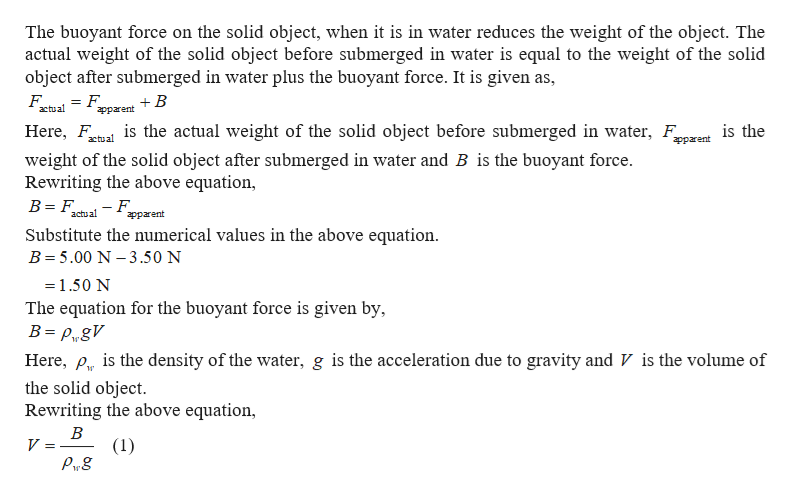Question
1 views

The gravitational force exerted on a solid object is 5.00 N as measured when the object is suspended from a spring scale as in Figure P9.26a. When the suspended object is submerged in water, the scale reads 3.50 N (Fig. P9.26b). Find the density of the object.

check_circle

Step 1help_outlineImage TranscriptioncloseThe buoyant force on the solid object, when it is in water reduces the weight of the object. The actual weight of the solid object before submerged in water is equal to the weight of the solid object after submerged in water plus the buoyant force. It is given as, = F. apparent F +B actual is the Here, F is the actual weight of the solid object before submerged in water, F actual apparent weight of the solid object after submerged in water and B is the buoyant force. Rewriting the above equation, actual фрrent Substitute the numerical values in the above equation. B= 5.00 N – 3.50 N =1.50 N The equation for the buoyant force is given by, B= P„gV Here, p. is the density of the water, g is the acceleration due to gravity and V is the volume of the solid object. Rewriting the above equation, (1) Prg fullscreen

### Want to see the full answer?

See Solution

#### Want to see this answer and more?

Solutions are written by subject experts who are available 24/7. Questions are typically answered within 1 hour.*

See Solution
*Response times may vary by subject and question.
Tagged in

### Fluid Mechanics